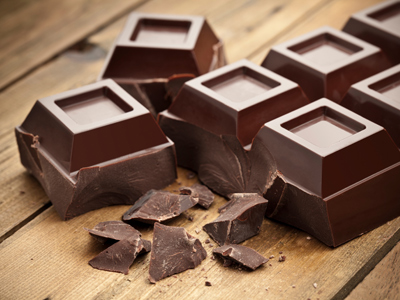What would be a suitable unit to measure the area of a chunk of chocolate?

# Perimeter and Area (Year 5)

In KS2 Maths, children learn about perimeter and area. In Year Five, they figure out how to find the perimeter of squares and rectangles. Now, they also explore other shapes like pentagons or hexagons and learn to calculate their area. Kids become confident with areas of squares and rectangles, understanding that square metres have 10,000 square cm, and square cm have 100 square mm.

The perimeter is the distance around a shape. To find a hexagon's perimeter, multiply one side's length by 6. For example, if the sides are 2 metres long, the perimeter is 12 metres. Area is like the size of a surface, important for planning how much paint a wall needs. Can you figure out the perimeter of a hexagon?

Test what you know about area and perimeter with this quiz for 9-10 year olds!

1.
What is the formula for calculating the perimeter of a regular hexagon?
4 x length of side
6 x length of side
6 x length + width
Length of side divided by 6
As a regular hexagon has 6 equal sides, just multiply the length of the sides by 6
2.

Which would be best measured in cm2?

Area of a playground
Area of a town
Area of a desk top
Area of a pen tip
Towns might be measured in km2, playgrounds in m2 and pen tips in mm2
3.
How many cm2 is equivalent to 1m2?
10cm2
100cm2
1,000cm2
10,000cm2
There are 100cm in 1m so 100 x 100 = 10,000
4.
What is the perimeter of a regular pentagon with sides of 5cm?
24cm
100cm
10cm
25cm
A pentagon has 5 sides, therefore 5 x 5cm will give you the perimeter
5.
Which would be a good estimate of the area of a classroom?
1,000mm2
100mm2
10m2
100m2
10m2 would be large enough for a room in a house but not big enough for a classroom
6.
A regular hexagon has a perimeter of 54cm. What is the length of the sides?
6cm
9cm
12cm
20cm
A regular hexagon has 6 equal sides. To calculate the length of each side, divide the perimeter by 6
7.
A rectangle has a perimeter of 38cm. The shortest sides are 7cm. What length are the two longest sides?
12cm
14cm
21cm
28cm
2 x 7 = 14
38 - 14 = 24
24 ÷ 2 = 12
8.
What is the formula for calculating the area of a rectangle?
2 x length x 2 x width
2 x length + 2 x width
Length + width
Length x width
If a rectangle measured 7cm by 10cm its area would be 70cm2
9.
What is the formula for calculating the perimeter of a rectangle?
2 x length x 2 x width
2 x length + 2 x width
Length + width
Length x width
2 sides of a rectangle are one length whilst the other two sides are a different length, for example 2 sides are 4cm long and the other 2 sides are 6cm long. This rectangle would have a perimeter of 20cm
10.
Which would be a suitable unit to measure the area of a chunk of chocolate?
Square grams
Square metres
Square millimetres
Square millilitres
Grams measure weight and millilitres measure volume. Square metres measure area but chocolate bars aren't that big!